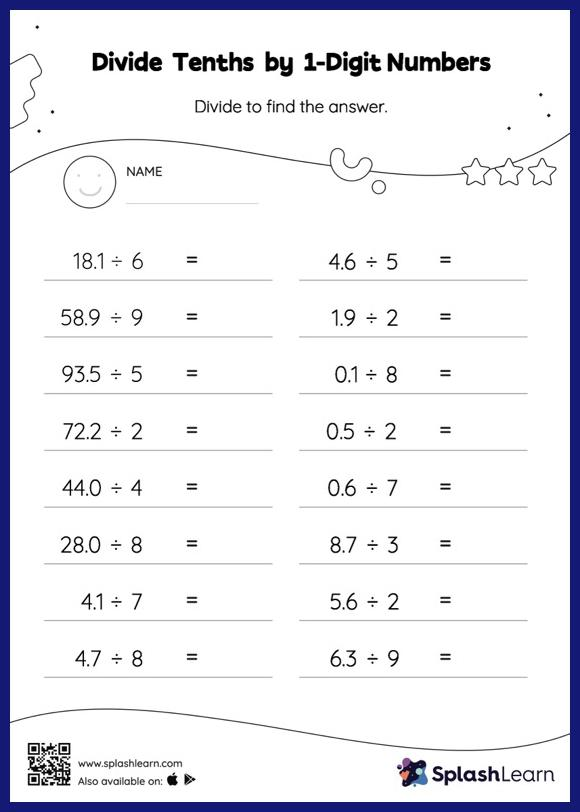# Divide Tenths by 1-Digit Numbers: Horizontal Division Worksheet

Home > Divide Tenths by 1-Digit Numbers: Horizontal DivisionThis worksheet consists of a set of questions on division where students are required to divide tenths by 1-digit numbers. This worksheet is about practicing with the horizontal format in which numbers are written side by side. To develop flexibility with numbers and operations, students need to have enough practice in this format and not just rely on the vertical/column method.